# How To Use IF…ELSE Statement In SQL Server

Posted May 10, 2019 by Vishwanath Dalvi in Database, SQL Server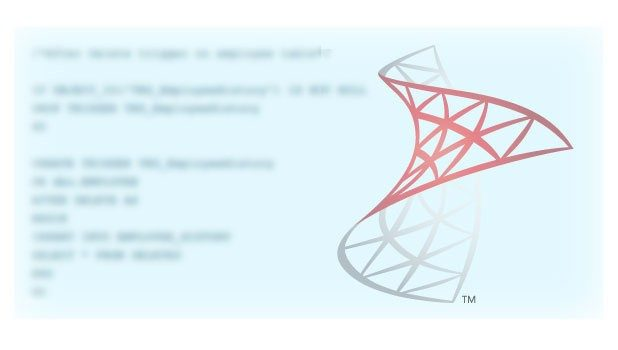A conditional statement IF…ELSE included in many programming languages. IF…ELSE works on boolean expression resulting in TRUE or FALSE. Besides, different actions can be performed on boolean expression results.

IF…ELSE in SQL Server is used to check expression and based on the results (TRUE or FALSE) execute another SQL statements block.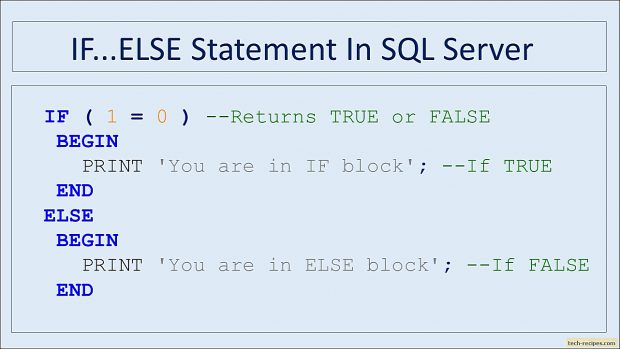### CASE vs IF Statement

Database developers get confused between CASE and IF statement. Therefore understanding the difference and use cases are important. Other programming languages use SWITCH statement instead of CASE.

1. IF is a logical statement to control the flow of batch whereas CASE statement determines the value to be used in a column.
2. CASE can be used within UPDATE/ SELECT/ ORDER BY to determine a value whereas IF can’t be used.

### BEGIN…END Block

BEGIN…END block executes the defined SQL statement in sequential order. As a result, this block combines statements and executes them in a fixed order.

```BEGIN
SQL Statement 1
SQL Statement 2
SQL Statement 3
END
```

### IF…ELSE Statement

Moreover, IF…ELSE works in T-SQL similarly to other programming languages. Let’s demonstrate the different use cases.

Note that, comparison within IF statement is done using = (single equal sign) in T-SQL unlike == (double equal sign) in other programming languages.

1.

### Single IF Statement

IF statement includes a single block of BEGIN…END. If the boolean condition returns TRUE then statements within BEGIN…END is executed. Otherwise, BEGIN…END block is completely skipped.

This example, string comparison evaluates to TRUE, therefore statements within a block are executed.

```IF ( 'Tech' = 'Tech' ) -- Returns TRUE Or FALSE
BEGIN -- Executed If TRUE
PRINT 'Test-1'
PRINT 'Test-2'
END -- Skipped If False
```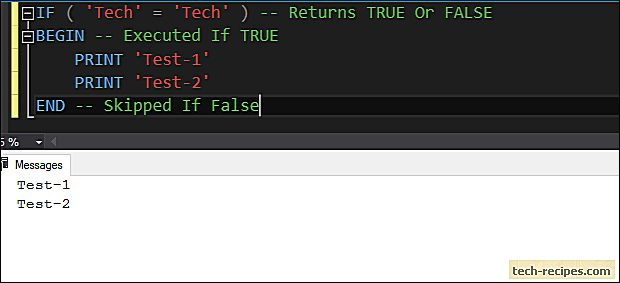In below example, comparison evaluates to false, therefore statements within a block are skipped.

```IF ( 1 = 0 ) -- Returns TRUE or FALSE
BEGIN -- Executed If TRUE
Print 'Test-1'
Print 'Test-2'
END
```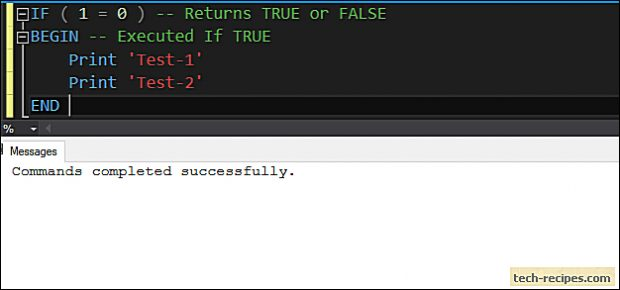In the following examples, demonstrating the use of variables within the IF statement. We can test for scalar or multiple value match with IN clause. If one of the value matches within IN clause then IF statement evaluates to TRUE.

```DECLARE @Year as INT = YEAR(GETDATE());

IF @Year = 2019
BEGIN
PRINT 'Yes, we are in 2019'
END
GO

DECLARE @Year as INT = YEAR(GETDATE());

IF @Year IN (2019, 2020, 2021) -- (@Year = 2019 OR @Year = 2020 OR @Year = 2021)
BEGIN
PRINT 'Yes, we are In';
END
```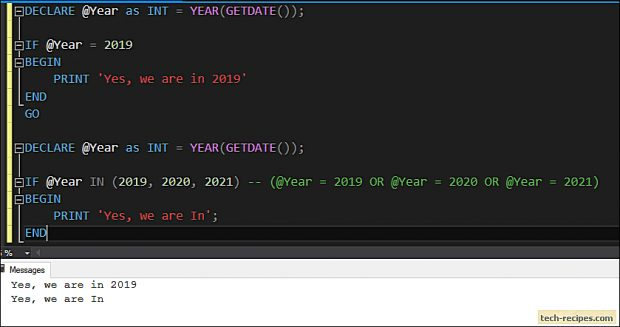2.

### Single IF…ELSE Statement

Demonstrating single IF…ELSE statement. If the condition evaluates to TRUE in the IF statement, then the statement within the first BEGIN…END block gets executed.

If the condition evaluates to FALSE then control is pass to ELSE block and second BEGIN…END block after ELSE is executed.

Following queries using IF…ELSE to evaluate a condition and if the result is TRUE then the first block of code is executed. Else the control is pass to ELSE block.

```IF ( 1 = 0 ) --Returns TRUE or FALSE
BEGIN -- If TRUE
Print 'You are in IF block'
END
ELSE -- If FALSE
BEGIN
PRINT 'You are in ELSE block'
END

DECLARE @Year as INT = YEAR(GETDATE());

IF @Year IN (2019, 2020, 2021) -- (@Year = 2019 OR @Year = 2020 OR @Year = 2021)
BEGIN
PRINT 'Yes, we are In';
END
ELSE
BEGIN
PRINT 'Skipped';
END
```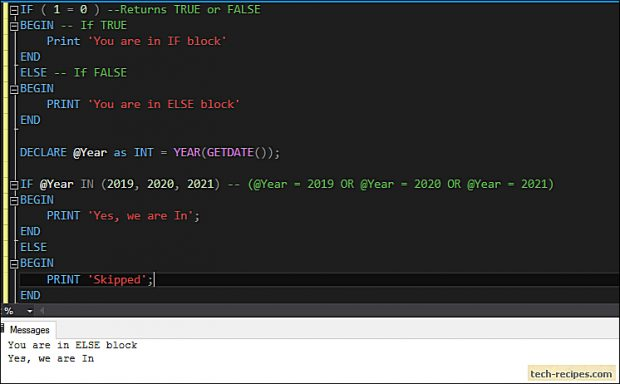3.

### Multiple IF…ELSE Statement

For many conditions we can use multiple IF…ELSE block with final ELSE (optional) statement. If any of the condition is evaluated to TRUE then other ELSE…IF and ELSE block is skipped. If none of the IF…ELSE block is TRUE then control is pass to final ELSE block.

In the below examples, we can see that second IF…ELSE block is evaluated to TRUE thus skipping all other conditions.

```DECLARE @Number as INT = 100;

IF (@Number = 101)
BEGIN
Print 'Number is 101'; --False
END
ELSE IF (@Number = 100)
BEGIN
Print 'Number is 100'; --True
END
ELSE IF (@Number = 102)
BEGIN
PRINT 'Number is 102'; --Skipped
END
ELSE
BEGIN
PRINT 'Number is out of scope'; --Skipped
END
```### Summary

Above all we have learned to use IF..ELSE conditional statement in SQL Server Including various examples. If you like this article you may like Tech-Recipes database archives to learn more useful stuff.